##全国校区# 20个能够有效提高 pandas数据分析效率的常用函数，附带解释和例子2020-08-07

pandas 是为了解决数据分析任务而创建的一种工具。pandas提供了大量能使我们快速便捷地处理数据的函数和方法，它是使Python成为强大而高效的数据分析环境的重要因素之一。今天小编就给大家分享一篇关于常见pandas函数的文章，希望对大家有所帮助。

pandas是一个受众广泛的python数据分析库。它提供了许多函数和方法来加快数据分析过程。pandas之所以如此普遍，是因为它的功能强大、灵活简单。本文将介绍20个常用的 Pandas 函数以及具体的示例代码，助力你的数据分析变得更加高效。``` import numpy as np
import pandas as pd```

# 1. Query

``` values_1 = np.random.randint(10, size=10)
values_2 = np.random.randint(10, size=10)
years = np.arange(2010,2020)
groups = ['A','A','B','A','B','B','C','A','C','C']
df = pd.DataFrame({'group':groups, 'year':years, 'value_1':values_1,'value_2':values_2})
df```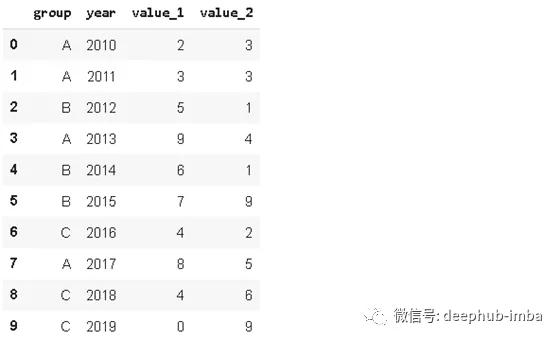` df.query('value_1 < value_2')`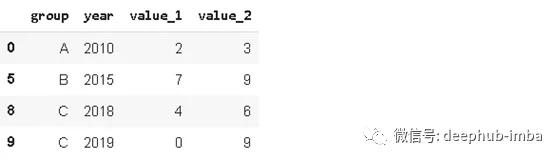# 2. Insert

``` # new column
new_col = np.random.randn(10)
# insert the new column at position 2
df.insert(2, 'new_col', new_col)

df```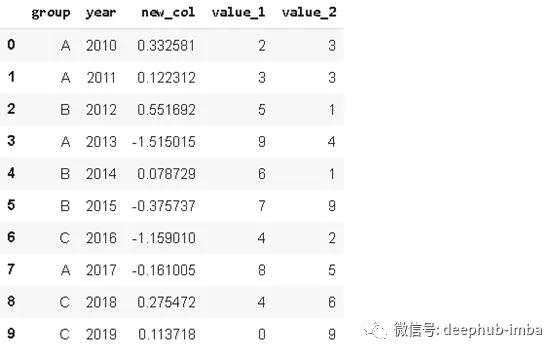# 3. Cumsum

``` df['cumsum_2'] = df[['value_2','group'].groupby('group').cumsum()]

df```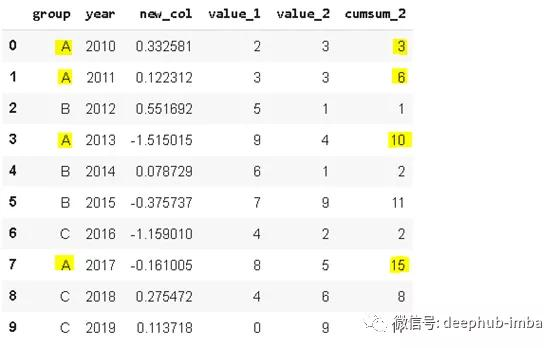# 4. Sample

Sample方法允许我们从DataFrame中随机选择数据。当我们想从一个分布中选择一个随机样本时，这个函数很有用。

``` sample1 = df.sample(n=3)
sample1```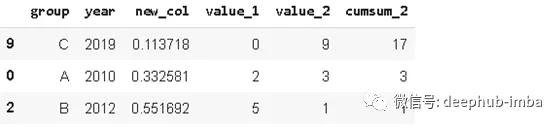``` sample2 = df.sample(frac=0.5)
sample2```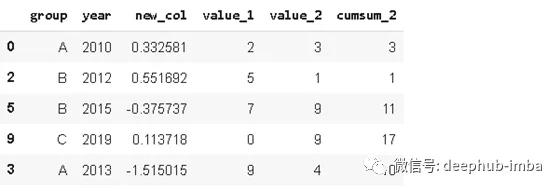# 5. Where

where函数用于指定条件的数据替换。如果不指定条件，则默认替换值为 NaN。

` df['new_col'].where(df['new_col'] > 0, 0)`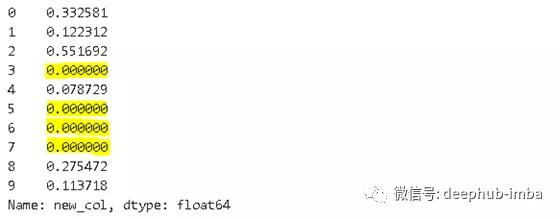where函数首先根据指定条件定位目标数据，然后替换为指定的新数据。上述代码中，where(df['new_col']>0,0)指定'new_col'列中数值大于0的所有数据为被替换对象，并且被替换为0。

``` df['new_col'].where(df['new_col'] > 0, 0)

np.where(df['new_col'] > 0, df['new_col'], 0)```

# 6. Isin

``` years = ['2010','2014','2017']
df[df.year.isin(years)]```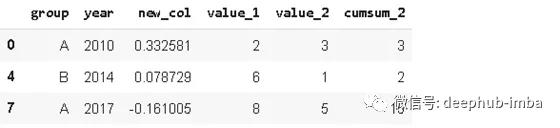# 7. Loc 和 iloc

Loc 和 iloc 函数用于选择行或者列。

• loc:通过标签选择
• iloc:通过位置选择

loc用于按标签选择数据。列的标签是列名。对于行标签，如果我们不分配任何特定的索引，pandas默认创建整数索引。因此，行标签是从0开始向上的整数。与iloc一起使用的行位置也是从0开始的整数。

` df.iloc[:3,:2]`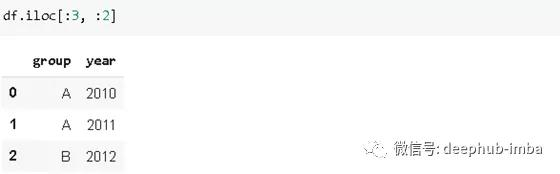` df.loc[:2,['group','year']]`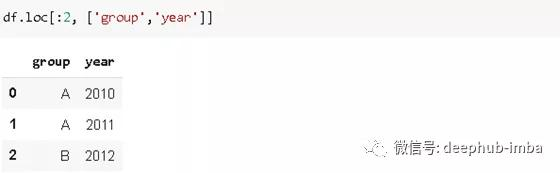` df.loc[[1,3,5],['year','value_1']]`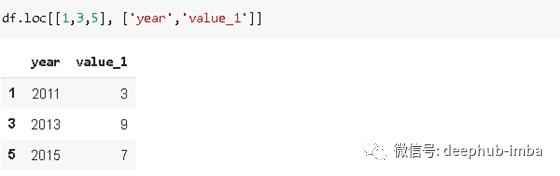# 8. Pct_change

` df.value_1.pct_change()`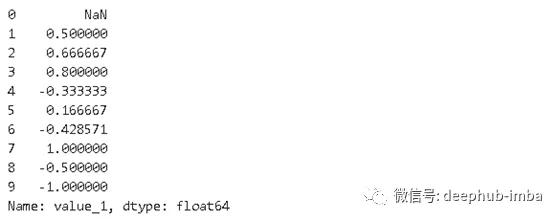# 9. Rank

Rank函数实现对数据进行排序。假设我们有一个包含[1,7,5,3]的序列。分配给这些值的等级为[1,4,3,2]。

``` df['rank_1'] = df['value_1'].rank()
df```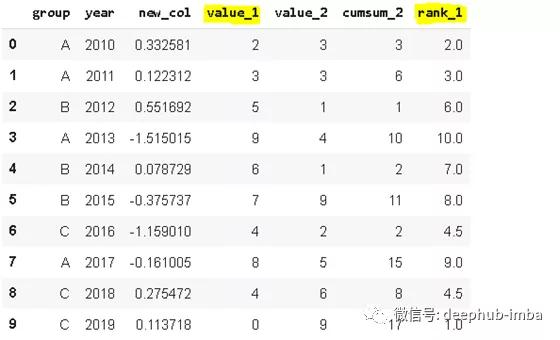# 10. Melt

Melt用于将维数较大的 dataframe转换为维数较少的 dataframe。一些dataframe列中包含连续的度量或变量。在某些情况下，将这些列表示为行可能更适合我们的任务。考虑以下情况：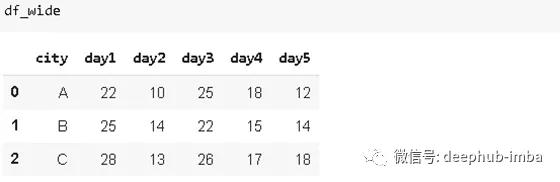``` df_wide.melt(id_vars=['city'])
df```# 11. Explode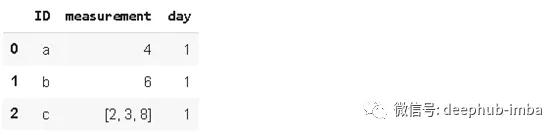``` df1.explode('measurement').reset_index(drop=True)
df```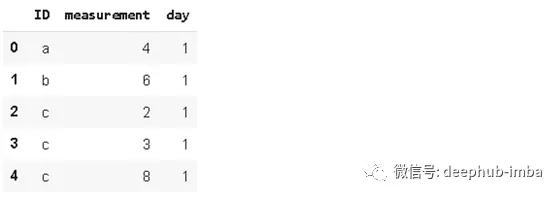# 12. Nunique

Nunique统计列或行上的唯一条目数。它在分类特征中非常有用，特别是在我们事先不知道类别数量的情况下。让我们看看我们的初始数据：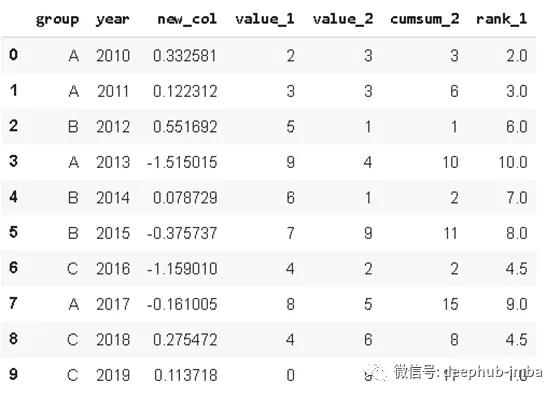``` df.year.nunique()
10
df.group.nunique()
3```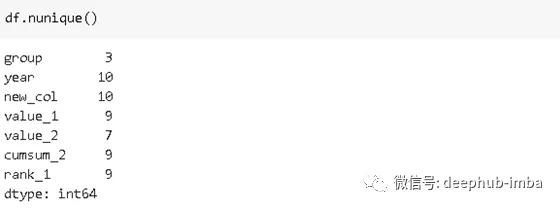# 13. Lookup

'lookup'可以用于根据行、列的标签在dataframe中查找指定值。假设我们有以下数据：``` df['Person_point'] = df.lookup(df.index, df['Person'])
df```# 14. Infer_objects

Pandas支持广泛的数据类型，其中之一就是object。object包含文本或混合（数字和非数字）值。但是，如果有其他选项可用，则不建议使用对象数据类型。使用更具体的数据类型，某些操作执行得更快。例如，对于数值，我们更喜欢使用整数或浮点数据类型。

infer_objects尝试为对象列推断更好的数据类型。考虑以下数据：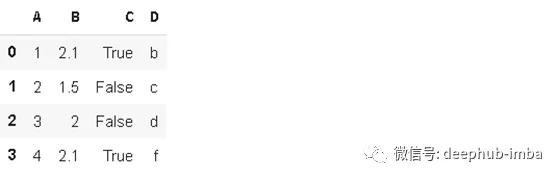``` df2.dtypes
A    object
B    object
C    object
D    object
dtype: object```

``` df2.infer_objects().dtypes
A      int64
B    float64
C       bool
D     object
dtype: object```

'infer_obejects'可能看起来微不足道，但在有很多列时作用巨大。

# 15. Memory_usage

Memory_usage()返回每列使用的内存量（以字节为单位）。考虑下面的数据，其中每一列有一百万行。

``` df_large = pd.DataFrame({'A': np.random.randn(1000000),
'B': np.random.randint(100, size=1000000)})
df_large.shape
(1000000, 2)```

``` df_large.memory_usage()
Index        128
A        8000000
B        8000000
dtype: int64```

``` df_large.memory_usage().sum() / (1024**2) #converting to megabytes

15.2589111328125```

# 16. Describe

describe函数计算数字列的基本统计信息，这些列包括计数、平均值、标准偏差、最小值和最大值、中值、第一个和第三个四分位数。因此，它提供了dataframe的统计摘要。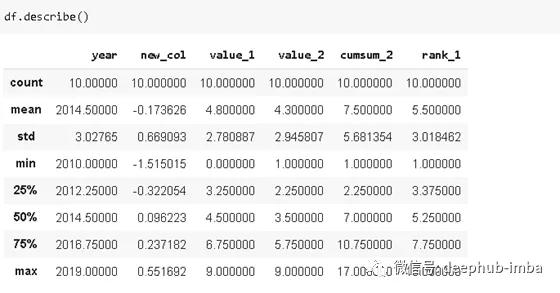# 17. Merge

Merge()根据共同列中的值组合dataframe。考虑以下两个数据: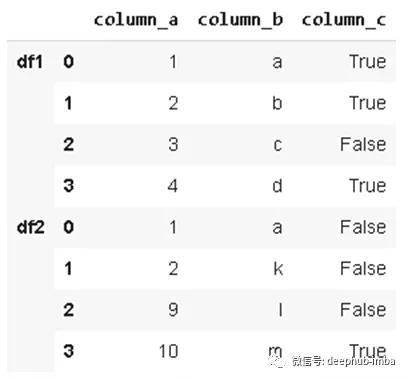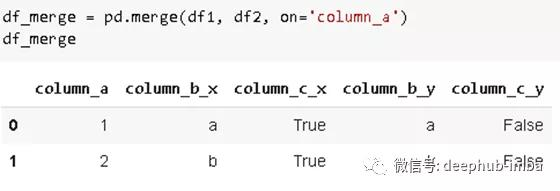df1和df2是基于column_a列中的共同值进行合并的，merge函数的how参数允许以不同的方式组合dataframe,如：“inner”、“outer”、“left”、“right”等。

• inner:仅在on参数指定的列中具有相同值的行（如果未指定其它方式，则默认为 inner 方式）
• outer:全部列数据
• left:左一dataframe的所有列数据
• right:右一dataframe的所有列数据

# 18. Select_dtypes

Select_dtypes函数根据对数据类型设置的条件返回dataframe的子集。它允许使用include和exlude参数包含或排除某些数据类型。

` df.select_dtypes(include='int64')`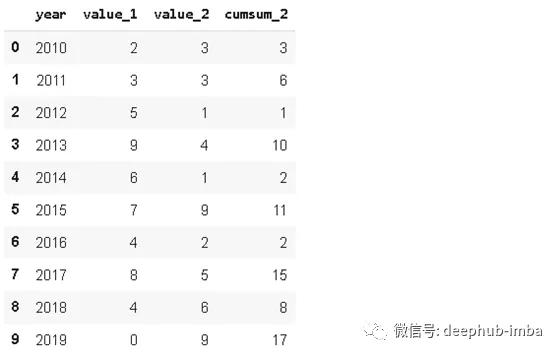` df.select_dtypes(exclude='int64')`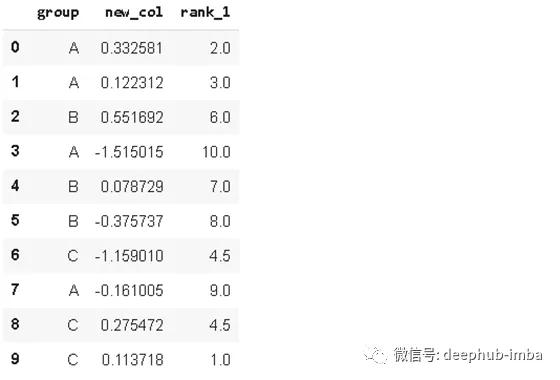# 19. Replace

` df.replace('A', 'A_1')`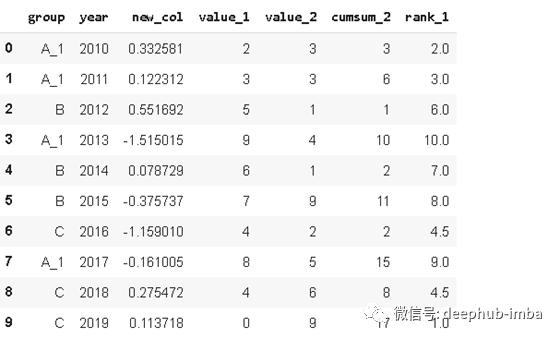` df.replace({'A':'A_1', 'B':'B_1'})`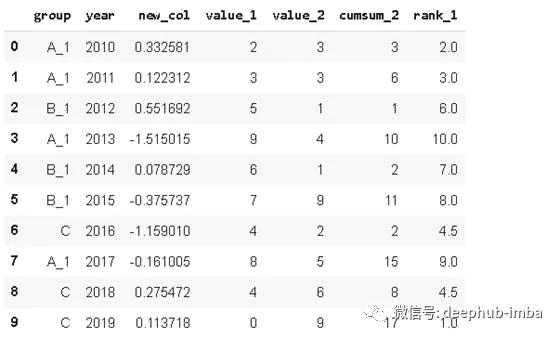# 20. Applymap

Applymap用于将一个函数应用于dataframe中的所有元素。请注意，如果操作的矢量化版本可用，那么它应该优先于applymap。例如，如果我们想将每个元素乘以一个数字，我们不需要也不应该使用applymap函数。在这种情况下，简单的矢量化操作（例如df*4）要快得多。

``` def color_negative_values(val):
color = 'red' if val < 0 else 'black'
return 'color: %s' % color```

` df3.style.applymap(color_negative_values)`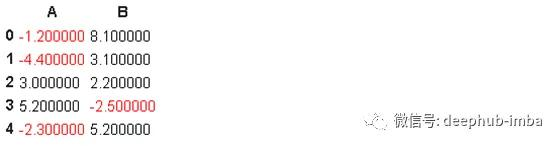deephub翻译组：Oliver Lee

OK